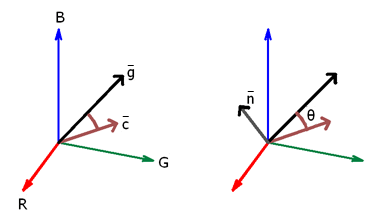Posted by: pixelero | November 5, 2008

## Flash Player 10: correcting color with Matrix3D and ColorMatrixFilter

The idea was to make a filter for removing a color cast from a picture – like in Photoshop you can by a single click convert some part to grayscale, and adjust the overall picture correspondingly – like the clouds in this picture:Mathematically I solved this by rotating the rgb-space so that the coordinates of the old color (c in illustration) are mapped to the grayscale-axis (g in illustration)- a diagonal line from 0,0,0 to 255,255,255.  To get the actual matrix for color transformation I first need to count a normal vector n, and angle between θ :Using new Vector3D and Matrix3D -classes, we can in fact do all this with just a few lines: here’s some essential part of code:

/* define the normal vector n = c x g  */
var normal:Vector3D = c.crossProduct(g);
normal.normalize();

/* theta, the angle between c and g, we need the value in degrees */
var theta:Number = Vector3D.angleBetween(c,g) *180.0/Math.PI;

/* create a new matrix3D, and apply such a rotation that point c transforms to the diagonal ‘greyscale’ line
from (0,0,0) to (255,255,255), r = g = b */

var mtrx:Matrix3D = new Matrix3D();
mtrx.prependRotation(-theta, normal);

/*  pass mtrx.rawData to the array in colormatrixfilter */

filter = new ColorMatrixFilter( [
mtrx.rawData,mtrx.rawData,mtrx.rawData, 0.0, mtrx.rawData, // red
mtrx.rawData,mtrx.rawData,mtrx.rawData, 0.0, mtrx.rawData, // green
mtrx.rawData,mtrx.rawData,mtrx.rawData, 0.0, mtrx.rawData, // blue
0.0, 0.0, 0.0, 1.0, 0.0 ] ); // alpha, no change

… and finally the Demo

( This could all have been done already with FP9, just that the calculations with vectors and matrices are so much simplier than with numeric variables,
matrix for rotating point(x,y,z) around (0,0,0)-(u,v,w) by angle θ,

( a small test for you: what’s the purpose of mtrx.rawData,  and  in above code, and what kind of values do they have ? )

## Responses

1.so that´s indeed very useful.

2.thx for sharing 😉

3. […] “Pixelero“로 가면 보다 자세한 내용을 알 수 있다. […]

4.Yes, very useful, thx for sharing. I really need it!

5.thanks for sharing, just what I need, am looking for similar functionality for flash Player 9 though, if you have a tip

Si